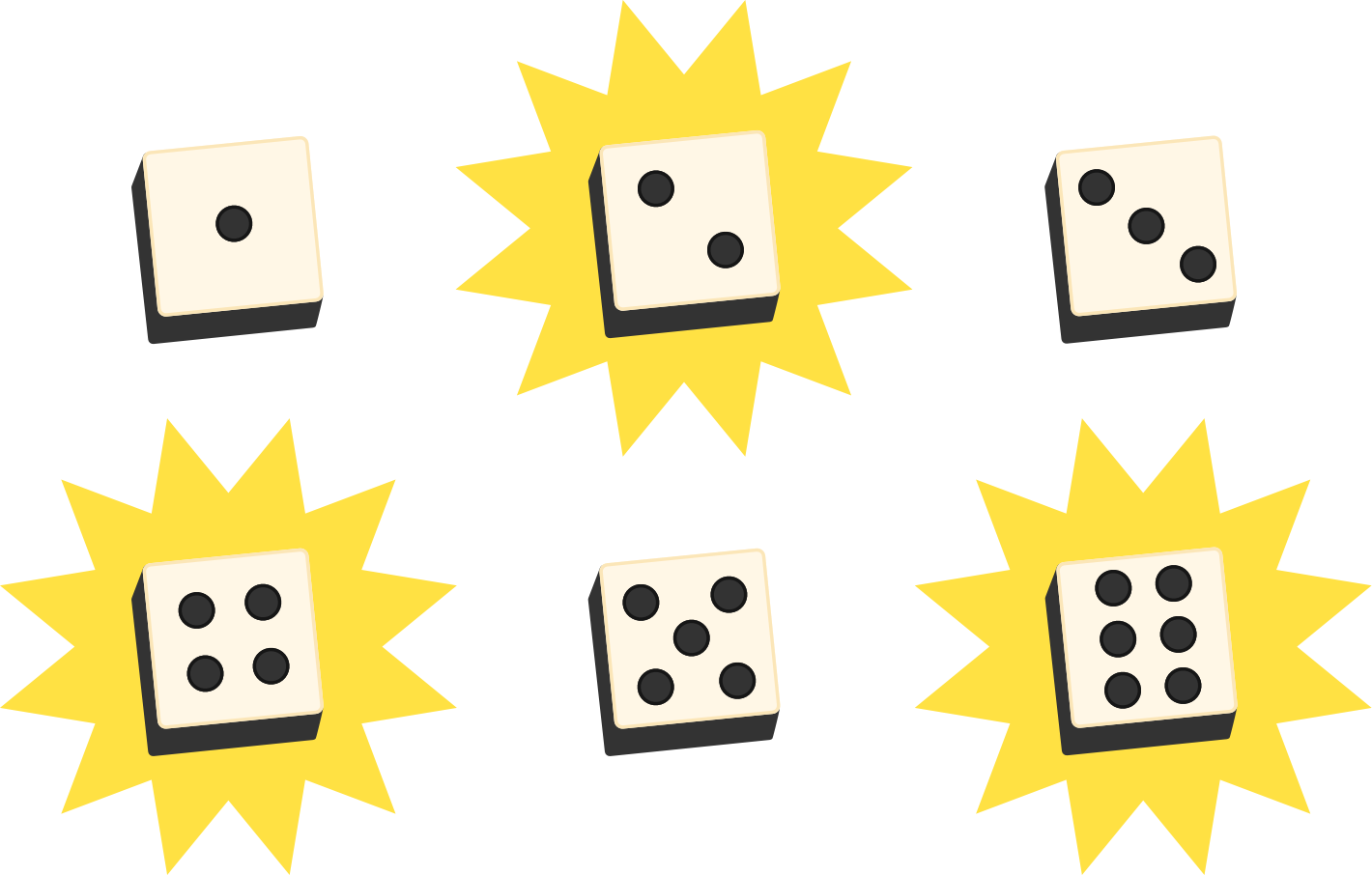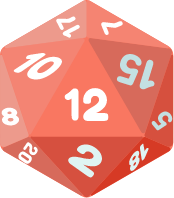### Applied Probability

The most straightforward probability calculation is "by outcomes". When there are some number of equally likely outcomes, the probability of a "successful" outcome can be calculated as $\frac{\text{\# of successful outcomes}}{\text{\# of total outcomes}}.$

For example, the probability of rolling an even number on a fair, six-sided die is $\frac{3}{6} = \frac{1}{2},$ since there are 6 equally likely outcomes (1, 2, 3, 4, 5, and 6) and 3 "successful" outcomes (2, 4, and 6).In this quiz, we'll look at a few examples where we can compute probabilities this way. From drug trials to winning raffle tickets, we'll find equally likely events to be important special cases in probability.

# Using Outcomes

You have signed up for an experimental drug trial, and you overheard the doctors say that all participants have been randomly assigned, with 400 people in the test group and 500 in the control group.

What is the probability that you are receiving the drug (that is, what is the probability that you're in the test group)?

# Using OutcomesA fair 20-sided die (as shown) is rolled. What is the probability that the result is a number divisible by 6?

# Using Outcomes

In the previous two questions, the groups of "total outcomes" and "successful outcomes" were fairly straightforward.

However, sometimes they can be less obvious, and it's important to ensure that you are counting outcomes which are actually equally likely. The next few questions explore this idea.

# Using OutcomesThere are 4 very large dormitories at your school that you could be assigned to. The three older dormitories each house the same number of students. The newest dorm houses twice the number of students as one of the older dorms.

What is the probability that you will be assigned to the newest dormitory, given that there is exactly one student assigned per dorm room, and dorm assignments are made simultaneously and at random?

# Using Outcomes

You are at a charity event and have purchased 1 ticket for a raffle.

Prior to the drawing, you are told that there are 200 people at the event (including yourself), and about $\frac{3}{4}$ of the people at the event purchased tickets to the raffle. Of those people, equal numbers of people purchased 1, 2, and 3 tickets, respectively.

Based on these estimations, and assuming only one winner, what is the probability that you win the raffle?

# Using OutcomesAshley and Allison want to go to the movies to see either Batman or Superman. They are willing to separate and go to different movies. Each of them independently flips a fair, standard coin to decide which movie they will go to.

What is the probability that they go to the same movie?

# Using Outcomes

Equally likely events are special but important cases in probability. By counting outcomes, we can assign numbers that measure the probability of witnessing specific results. Counting strategies are therefore very important in the study of probability.

In the next quiz on probability applications, we look back at what we covered so far in this chapter and also get a glimpse of what lies ahead.

×